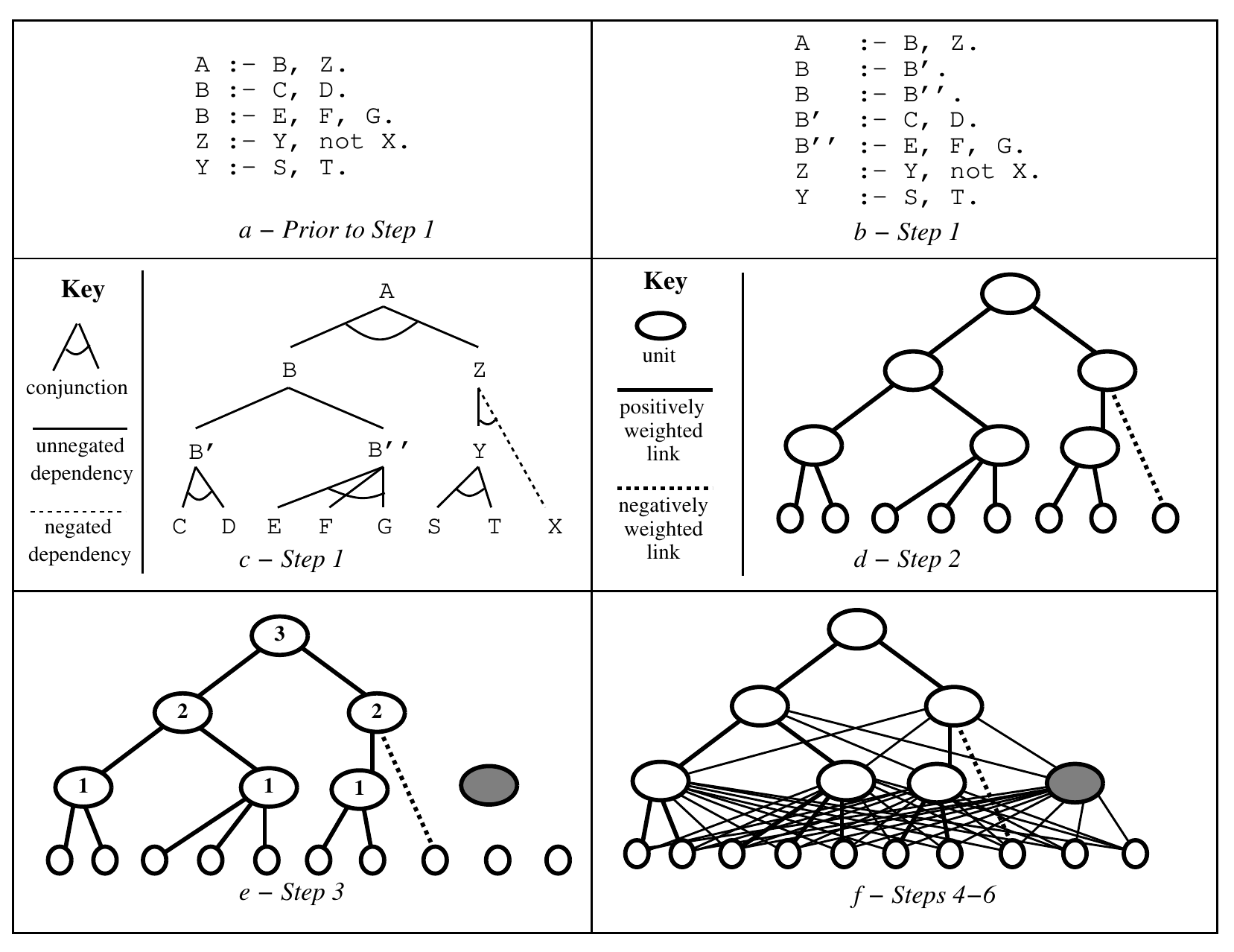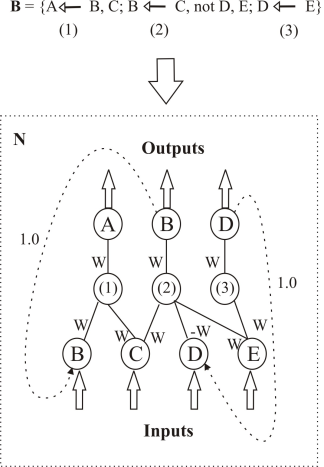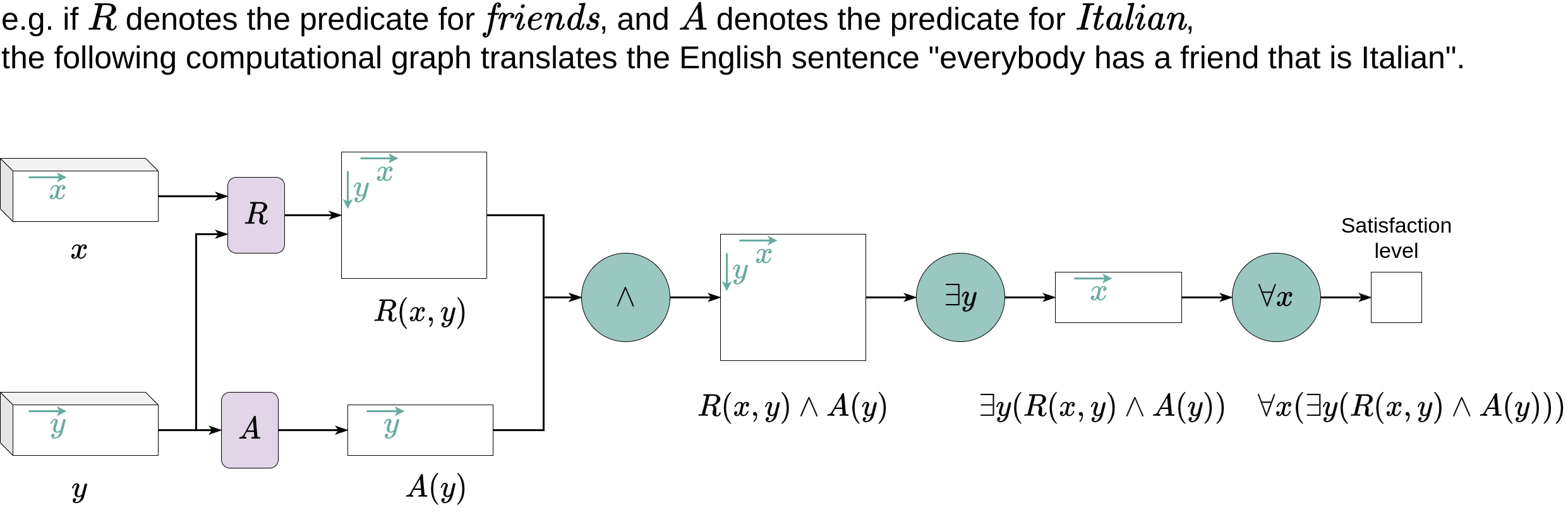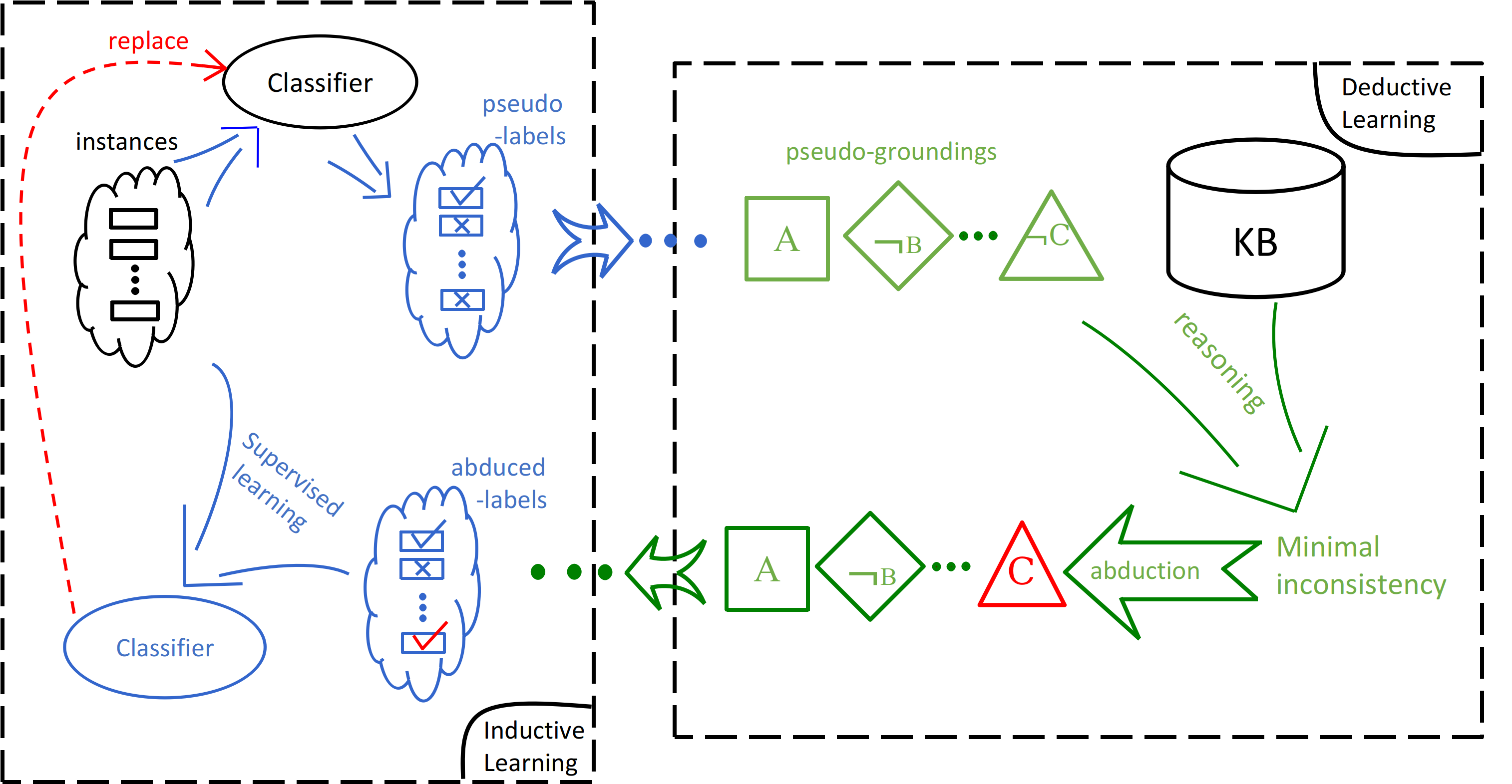# 符号学习简介

(Press ? for help, n and p for next and previous slide)

2022年-春季

https://daiwz.net### 路径图## 神经符号学习

1. KBMC
2. Embedding 与命题化
3. 特殊神经模块
4. 混合模型### 另一种 KBMC

Knowledge-Based Model Construction where $$model=NN$$：构建具有逻辑结构的神经网络模型

\begin{eqnarray*} h_1 &\leftarrow& i_1 \wedge i_2 \wedge i_3\\ h_2 &\leftarrow& i_1 \wedge i_2 \wedge i_3\\ &\ldots&\\ o_2 &\leftarrow& h_1 \wedge h_2 \wedge h_3 \wedge h_5\\ o_3 &\leftarrow& h_1 \wedge h_2 \wedge h_3 \wedge h_5 \end{eqnarray*}### KBANN

Knowledge-Based Artificial Neural Networks [Towell & Shavlik, 1994]

1. 专家系统（Hand-built classifiers）：
• 知识的完备性与可靠性难以保证
• 背景知识过于复杂，导致推理非常困难（intractable）
• 人工编写的知识难以改变
2. 机器学习（Empirical learning）：
• 特征选择对模型性能影响过大
• 不同任务需要使用不同的特征
• 特征构建（在原始特征基础上生成新特征）风险高
• 仅仅关注统计意义上的“大部分”，泛化时容易忽略 outliers### KBANN

• 将逻辑表达式转化为一个 MLP
• body literals 对公式的影响相同，因此只有一个权重### KBANN

1. 转换析取式，例如：

\begin{eqnarray*} A& \leftarrow& B, C, D.\\ A& \leftarrow& D, E, F, G. \end{eqnarray*}

转换为：

\begin{eqnarray*} A&\leftarrow& A'\\ A'& \leftarrow& B, C, D.\\ A&\leftarrow& A''\\ A''& \leftarrow& D, E, F, G. \end{eqnarray*}
2. 将规则转换为神经网络
3. 添加隐层节点（可选）
4. 添加神经元连接### 逻辑程序并行计算模型

Parallel Computational Model for Logic Programming [Hölldobler & Kalinke, 1994]

1. 非定子句（definite clause）构成的逻辑理论 $$P$$ 对于 immediate consequence 算子 $$T_P$$ 可能不存在不动点，其语义无法定义。
2. 将 $$T_P$$ 算子延拓为一个完备测度空间（如 Banach 空间）上的映射，即可保证它存在不动点。

1. 与 KBANN 类似，命题原子表示神经元；
2. 区别在于，每个公式是一个隐层节点；

• 节点内的数值为激活权重；### CI$$^2$$LP

The Connectionist Inductive Learning and Logic Programming System [d’A. Garcez & Zaverucha, 1999]

1. 定义两种激活阈值：
• $$0< A_{\text{min}}< 1$$：当神经元取值 $$A\geq A_{\text{min}}$$ 时输出 $$true$$；
• $$-1 < A_{\text{max}}< 0$$：当神经元取值 $$A\leq A_{\text{max}}$$ 时输出 $$false$$；
2. 逻辑子句（长度 $$k_l$$）隐层神经元激活阈值 $$\theta_l$$ ： $\theta_l=\frac{(1+A_{\text{min}})(k_l-1)}{2}W$
3. 逻辑命题（定子句数 $$\mu_l$$）神经元激活阈值 $$\theta_A$$ ： $\theta_A=\frac{(1+A_{\text{min}})(1-\mu_l)}{2}W$
4. 隐层采用 sigmoid 激活函数，命题原子采用线性激活函数。### CILP++

CILP with Bottom Clause Propositionalization [França, et. al., 2014]

1. 利用 Progol 的 mode 语言定义背景知识；
2. 通过逆蕴涵或其他方式构建底子句（bottom clauses）
3. 将底子句中的原子与子句分别作为神经元与隐层节点，用类似 CI$$^2$$LP 的方式构建神经网络；
4. 将所有样本命题化为向量，并进行学习。### 神经网络中的符号规则抽取## 神经符号学习

1. KBMC
2. Embedding 与命题化
3. 特殊神经模块
4. 混合模型### 基于嵌入式表示的方法

1. 基本放弃符号表示，将符号表示在 embedding space
2. 仅仅采用 embedding space 中的向量（张量）运算来推理
3. 利用 Fuzzy operators 来近似逻辑演算### Neural Tensor Network

Reasoning with neural tensor networks for knowledge base completion [Socher et. al., 2013]### NTN 的训练

\begin{equation*} J(\Theta)=\sum_{i=1}^N\sum_{c=1}^C\max(0,1-g(T^{(i)})+g(T_c^{(i)}))+\lambda||\Theta||^2_2 \end{equation*}

\begin{equation*} g(e_1,r,e_2)= u_r^T\tanh\left(h+M_r\left[ \begin{array}{l} v_{e_1}\\ v_{e_2} \end{array} \right]+b_r\right) \end{equation*}
• $$v_{e_i}\in\mathbb{R}^d$$ 实体 $$e_i$$ 的 embedding
• $$h=v_{e_1}^TW_r^{[1:k]}v_{e_2}$$ 将三元组 embedding 通过双线性（bilinear）映射成一个向量
• $$W_r^{[1:k]}\in\mathbb{R}^{d\times d\times k}$$ 关系 $$r$$ 的embedding
• $$u_r\in\mathbb{R}^k$$ 、 $$M_r\in\mathbb{R}^{k\times 2d}$$ 和 $$b_r\in\mathbb{R}^k$$ 均为神经网络中与 $$r$$ 相关的参数 $$\Theta_r$$### Logic Tensor Network

LTN [Badreddine, et. al., 2022] 试图将 NTN 扩展为更完整的 FOL 语言。

• Grounding $$p(e_1, \ldots, e_k)$$ 的计算方式与 NTN 类似： $\mathcal{G}(p(e_1, \ldots, e_k))=\mathbf{v}W_p \mathbf{v}^T$
• $$W_p$$ 为谓词 $$p/k$$ 的 embedding
• $$\mathbf{v}=(v_{e_1}, \ldots, v_{e_k})$$ 是 $$k$$ 个 embedding 向量的拼接### Logic Tensor Network

LTN [Badreddine et. al., 2022] 试图将 NTN 扩展为更完整的 FOL 语言。

• 对于含有逻辑连词的公式，用 fuzzy operators 来近似其推理模式### Logic Tensor Network

LTN [Badreddine et. al., 2022] 试图将 NTN 扩展为更完整的 FOL 语言。### Logic Tensor Network

LTN [Badreddine et. al., 2022] 试图将 NTN 扩展为更完整的 FOL 语言。

\begin{equation} \mathop{\arg\max}\limits_{\Theta}\mathcal{G}(R_1\wedge\ldots\wedge R_n;\Theta) \end{equation}### Neural Theorem Prover

NTP[Rocktäschel et. al., 2015] 试图模仿 SLD-Resolution（称为 backward chaining）实现推理。

1. 与 KBANN 类似，用逻辑规则初始化（编译）网络结构
• 用 metarule 初始化则可达到归纳和谓词发明的效果
• 需要定义最大递归深度 $$d$$
2. 用神经网络 $$\mathtt{unify}_\theta(A,B,S)=S'$$ 实现合一操作
• $$A$$ 与 $$B$$ 为待合一的两个原子公式（embedding 拼接向量）
• $$S$$ 与 $$S'$$ 分别为合一进行之前/之后的证明状态
• 包含合一操作中使用的替换、成功概率等等
3. 用神经网络 $$\mathtt{AND}$$ 与 $$\mathtt{OR}$$ 实现和取和析取### 命题化 vs Embeddings

Propositionalization and Embeddings: Two Sides of the Same Coin [Lavrac et. al., 2020]

• 命题化：用关系型特征将知识库转化为表格数据（one-hot）
• 每个维度代表一个符号
• Embedding：将符号表示在一个连续度量空间中
• 符号表示分散在不同维度中### TensorLog

TensorLog: A Differentiable Deductive Database [Cohen, 2016]

• 常量 $$c_i\in\mathcal{C}$$ 表示为 one-hot 向量 $$\mathbf{u}_c$$
• 二元谓词 $$r$$ 则用一个稀疏矩阵 $$\mathbf{M}_r$$ 表示
• $$\mathbf{M}_r^{i,j}=1$$ 当且仅当 $$r(c_i, c_j)=true$$
• 一元谓词退化为一个向量 $$\mathbf{v}_r$$
• 原子公式 $$x=r(a,b)$$ 的赋值 $\mathbf{v}_a^T\mathbf{M}_r\mathbf{v}_b$### TensorLog

TensorLog: A Differentiable Deductive Database [Cohen, 2016]

• 可以对带权的链式规则进行推理 
• 求解 $$r(Y,X)\leftarrow s(Y,Z)\wedge t(Z,Y)$$ 当 $$X=x$$ 时 $$r(Y,x)$$ 对哪些 $$Y$$ 成立 $\mathbf{s}(x)=\mathbf{M}_{s}\cdot\mathbf{M}_{t}\cdot\mathbf{v}_x$
• 求解上式对某个 $$Y=y$$ 是否成立 $\text{score}(y|x)=\mathbf{v}_y^T\cdot\mathbf{s}(x)$### $$\partial$$ILP

$$\partial$$ILP [Evans & Grefenstette, 2018] 更进一步地将元解释学习（MIL）的方式表示为可微分计算

1. 利用 $$H^2_2$$ meta-rule 将所有可能的候选模型构建出来
2. 用 one-hot 向量表达空间状态
3. 用神经网络来近似 immediate consequence 算子
4. 用 fuzzy operators 进行逻辑演算### Neural Logical Machine

TensorLog、$$\partial$$ILP 只能 考虑 2 元谓词，而 NLM [Dong et. al., 2020] 对此作出改进：

• Expansion

validMove(X,Y) :- movable(X), placeable(Y). %% 维度不匹配
%%% Expansion
movableX(X,Z) :- movable(X).
placeable(Y,Z) :- placeable(Y).
validMove(X,Y) :- movable(X,Y), placeable(Y,X). %% 维度匹配

• Reduction

movable(X) :- \+isGround(X),\+on(Y,X). %% 维度不匹配
%%% Reduction
clear(X) :- \+on(Y,X).
movable(X) :- \+isGround(X),clear(Y). %% 维度匹配### Neural Logical Machine

TensorLog、$$\partial$$ILP 只能 考虑 2 元谓词，而 NLM [Dong et. al., 2020] 对此作出改进：

• 以上两种算子将一条规则内的谓词矩阵 $$\mathbf{M}$$ 统一成相同维度## 神经符号学习

1. KBMC
2. Embedding 与命题化
3. 特殊神经模块
4. 混合模型### Memory Network

MemNN [Weston et. al., 2015] 在神经网络中添加 memory 模块

1. 记忆体 $$\mathbf{m}$$ 拥有多个 slot $$\mathbf{m}_i$$
2. $$I$$ (Input feature map) 将输入信号转换 $$x$$ 为 embedding $$I(x)$$
3. $$G$$ (Generalisation) 用来更新 $$\mathbf{m}_i=G(\mathbf{m}_i, I(x), \mathbf{m})$$
4. $$O$$ (Output feature map) 根据输入和记忆体产生输出 $$o=O(I(x),\mathbf{m})$$
5. $$R$$ (Response) 对输出进行解码 $$r=R(o)$$### NTM 与 DNC

Neural Turing Machine [Graves et. al., 2014] 与 Differential Neural Computer [Graves et. al., 2016] 与 MemNN 类似，通过外部存储和读写头来实现复杂推理。

• $$t$$ 时刻的 memory 状态 $$\mathbf{m}^t$$
• 读取结果 $$\mathbf{r}^t=\sum_i w^t_i\mathbf{m}^t_i$$，$$\mathbf{w}^t$$ 为读取权重
• NTM 不区分读写权重，倾向于对局部进行集中读写，容易覆盖重要内容
• DNC 区分读写，动态地分配读写地址，引入时序管理### NALU

Neural Arithmetic Logic Units [Trask et. al., 2018]

• 动机： 神经网络难以在算数问题上泛化
• 目标： 学习算数函数，例如• 方案：引入特殊的神经元来近似算数运算### 神经排序

Neural Sort [Grover et. al., 2019]

• 动机：排序是一种不可微运算
• 目标• 方案：利用排列矩阵 $$P_{\mathbf{z}}$$ 来实现可微的近似排序模块
\begin{align} L(\theta, \mathbf{s})&=f(P_{\mathbf{z}};\theta)\\ \text{where } \mathbf{z} &=\mathtt{sort}(\mathbf{s}), \\ \text{e.g., }P_{[1,4,2,3]}&=\begin{pmatrix} 1 & 0 & 0 & 0\\ 0 & 0 & 0 & 1\\ 0 & 1 & 0 & 0\\ 0 & 0 & 1 & 0 \end{pmatrix} \end{align}## 神经符号学习

1. KBMC
2. Embedding 与命题化
3. 特殊神经模块
4. 混合模型### 两种AI范式

• 深度神经网络、统计学习为代表的当下主流人工智能；
• 主要通过积累数据（样本）来提升模型性能；
• 直觉、捷径、唯一答案、速度快（System 1）；

• 逻辑推理、专家系统为代表的传统人工智能；
• 主要通过添加符号表达的知识（程序）来提高搜索效率；
• 推理、逐步、允许歧义、速度慢（System 2）；

Y. Bengio. From System 1 Deep Learning to System 2 Deep Learning. Keynote at NeurIPS 2018.### 符号—神经混合系统### DeepProbLog

[Manhaeve et. al., 2018]• 将基于半环的 AProbLog 与神经谓词结合
• ProbLog 部分负责推理、神经网络负责感知### 反绎学习（ABL）### ABL 问题形式化训练样本：$$\langle x,y\rangle$$

1. 机器学习 （例如，神经网络）： $z=f(x;\theta)=\text{Sigmoid}(P_\theta(z|x))$
• 学习一个感知函数：sensory input ($$x$$) ⟶ logic symbols ($$z$$);
2. （概率）逻辑推理 （例如，（概率）程序）： $B\cup z\models y$
• 先验知识（例如，程序、知识图谱） $$B$$;
• 推理出最有可能的符号 $$z$$ 以训练感知函数令 $$z=f(x)$$
3. 优化：最大化 $$z\cup f$$ 关于 $$\langle x, y\rangle$$ 和 $$B$$ 的一致性（例如，后验概率 $$\text{Pr}(z,f\mid x,y,B)$$）.### 混合模型的主要挑战